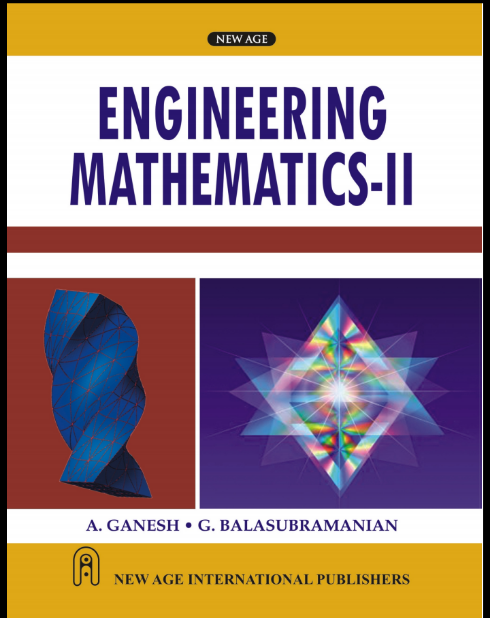### Engineering Mathematics 2Engineering, Mathematics, Maths,

The book contains eight chapters, and each chapter corresponds to one unit of the syllabus. The topics covered are: Unit I and II— Differential Calculus, Unit III and IV—Integral Calculus and Vector Integration, Unit V and VI— Differential Equations and Unit VII and VIII—Laplace Transform